## ML Aggarwal Class 7 Solutions for ICSE Maths Chapter 4 Exponents and Powers Ex 4.2

Question 1.
Using laws of exponents, simplify and write the following in the exponential form: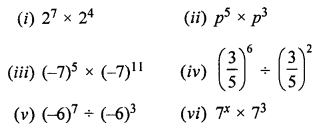Solution:Question 2.
Simplify and write the following in the exponential form:
(i) 53 × 57 × 512
(ii) a5 × a3 × a7
(iii) (712 × 73) ÷ 74
Solution:
(i) 53 × 57 × 512 = 53+7+12 = 522
{∵ am × an × ap = am+n+p}
(ii) a5 × a3 × a7 = a5+3+7 = a15
(iii) (712× 73) ÷ 74 = 712+3 ÷ 74 = 715 – 74 = 715-4 = 711

Question 3.
Simplify and write the following in the exponential form:
(i) (22)100
(ii) ((-7)6)5
(iii) (32)5 × (34)7
Solution:
Using (am)n = amn
(i) (22)100 = 22×100 = 2200 {(am)n = amn}
(ii) [(-7)6]5 = (-7)6×5 = (-7)30
(iii) (32)5 × (34)7 = 32×5 × 34×7 = 310 × 328 = 310+28 = 338

Question 4.
Simplify and write in the exponential form: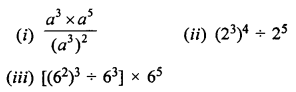Solution:Question 5.
Simplify and write in the exponential form:Solution: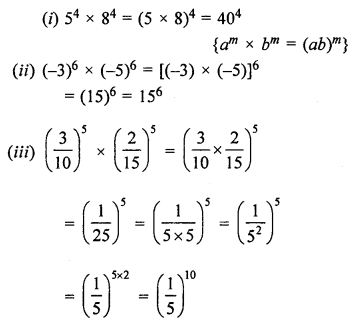Question 6.
Simplify and express each of the following in the exponential form: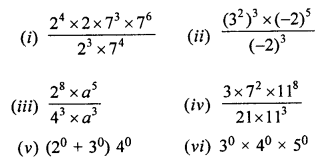Solution: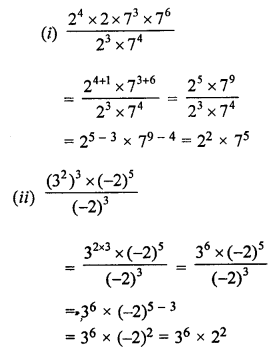Question 7.
Express each of the following rational numbers in the exponential form: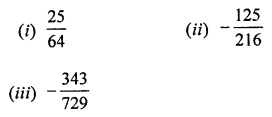Solution:Question 8.
Simplify the following:Solution:Question 9.
Simplify the following: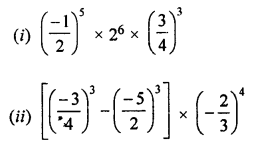Solution:Question 10.
Simplify the following: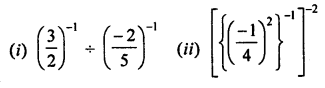Solution:Question 11.
Simplify: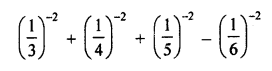Solution:Question 12.
Express each of the following as a product of prime factors in the exponential form:
(i) 108 × 192
(ii) 729 × 64
(iii) 384 × 147
Solution: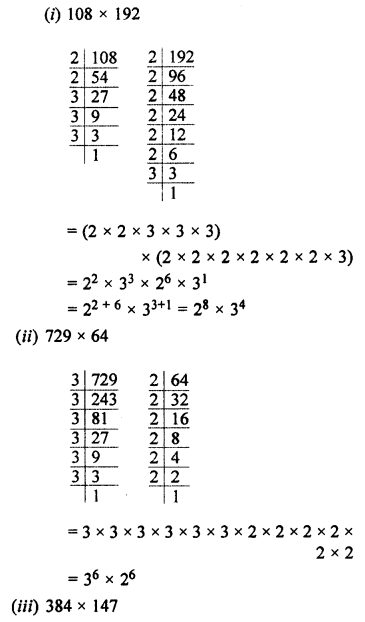Question 13.
Simplify and write the following in the exponential form:
(i) 33 × 22 + 22 × 50
(ii) 92 + 112 – 22 × 3 × 170
Solution:Question 14.
(i) By what number should we multiply 34 so that the product is 37?
(ii) By what number should be multiple (-6)-1 so that the product is 10-1?
Solution:
(i) Product = 37
Required number = 37 ÷ 34 = 37-4 = 33 = 27
(ii) Product = 10-1
Required number = 10-1 ÷ (-6)-1Question 15.Solution: